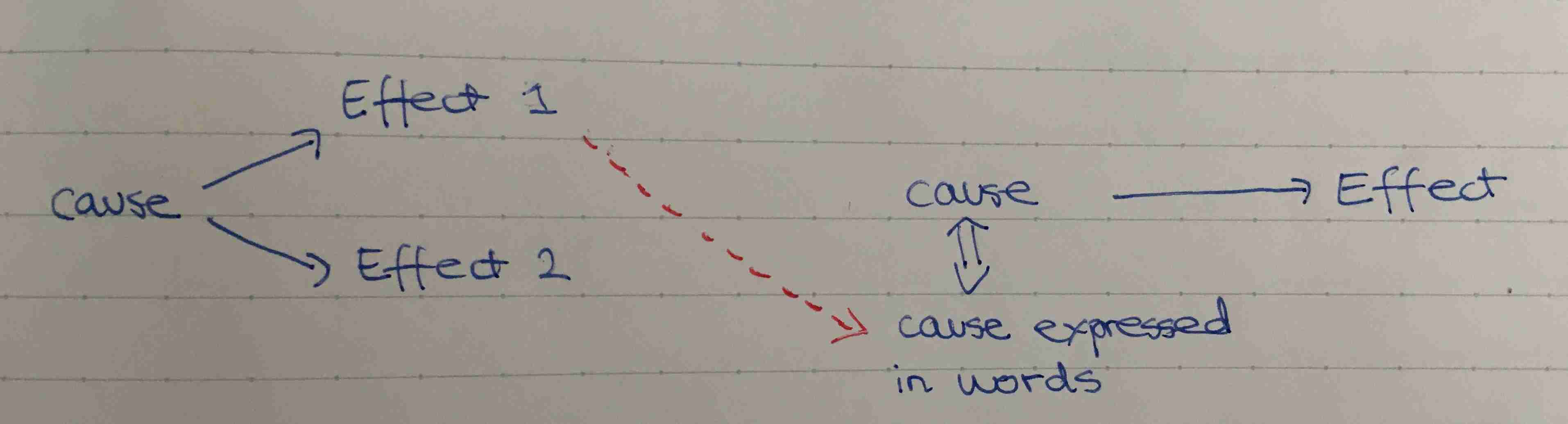Show Question
Math and science::Analysis::Tao::08. Infinite sets

# Series on uncountable sets (a special case)

We have a definition for series on countable sets (defined as a sum remapped over the natural numbers). In general, this definition doesn't extend to uncountable sets; however, there is a special case where we can make this extension.

Note: the following definitions have a flaw that is examined on the flip side. Understanding the flaw is probably the highlight of this card.

Let $$X$$ be a set (which could be uncountable), and let $$f : X \rightarrow \mathbb{R}$$ be a function. Consider every subset of $$X$$ and consider the sum over each of these subsets. The set of all of these sums—if its supremum is less than $$\infty$$, then the set $$\{x \in X: f(x) \ne 0\}$$ is at most countable*. In this case, we make the definition

$$\sum_{x \in X}f(x)$$ is absolutely convergent, and the sum is given by:

$\sum_{x \in X}f(x) := \sum_{x \in X: f(x) \ne 0} f(x)$

So, we have found a case where it makes sense to represet the sum over an uncountable set as a sum over a countable one.

*This requires the axiom of choice.

### More precisely

It is good to clean up the above definition so as to make clear what is an if and what is an iff relationship—we need to reshuffle the presentation. The next presentation uses the order given by Tao.

Firstly, we want to be able to refer to the antecedent of the first conditional. To give a name to the antecedent we make an iff definition:

We say that the series $$\sum_{x \in X}f(x)$$ is absolutely convergent iff

$\sup \left\{ \sum_{x \in A} |f(x)| : A \subseteq X, A \text{ is finite} \right\} < \infty$

We then say that, if the series $$\sum_{x \in X}f(x)$$ is absolutely convergent, then the set $$\{ x \in X: f(x) \ne 0 \}$$ is at most countable and we define the value of $$\sum_{x \in X}f(x)$$ by:

$\sum_{x \in X}f(x) := \sum_{x \in X: f(x) \ne 0} f(x)$

This presentation avoids two potential problems:

1. Not having the convergence of the series $$\sum_{x \in X}f(x)$$ imply that the set $$\{ x \in X: f(x) \ne 0 \}$$ is countable.
2. Not having the convergence of the series $$\sum_{x \in X}f(x)$$ imply that the above supremum is finite.

The below image shows how the two presentations differ in what they are describing.p192